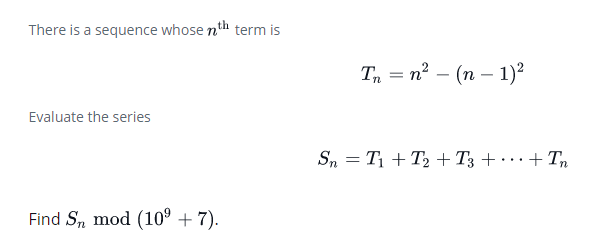# Summing the N series

This code doesn’t pass all the tests. Can you help please ?

``````function summingSeries(n) {
return n**2 % (10**9 + 7);
}
``````Which tests are failing?

They are hidden tests. Mathematically everything is correct but the code still doesn’t pass some tests Maybe the problem is in how javascript handle big numbers MAYBE

The math looks ok to me, so I’d guess a big number problem too.

What’s the problem here ? In Python the same code works very well

How to solve the issue of big numbers in javascript ?

Have you looked at the MDN page on BigInt?

You could try reducing n mod 10**9 + 7 before squaring it.

I’ve tried both methods
I used BigInt but It doesn’t work maybe because the compiler doesn’t support it.
I also tried to do the calculation before squaring n as you see in the code below

``````function summingSeries(n) {
var m = 10**9 + 7;
return ((n%m) * (n%m)) % m;
}
``````

Well, I solved this problem using Python. If the topic of big numbers has no importance in Javascript, I will go to something else

Big numbers are important in every language. I have no idea what the test cases are though, so I can only blindly guess at what might be happening.

1 Like